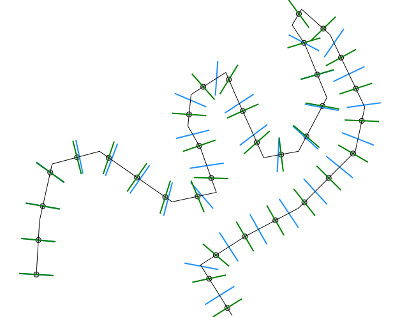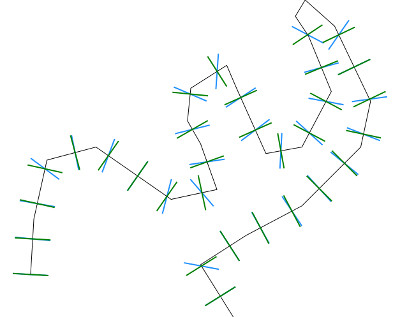## NAME

v.transects - Creates transect lines or quadrilateral areas at regular intervals perpendicular to a polyline.

vector, transect

## SYNOPSIS

v.transects
v.transects --help
v.transects [-l] input=name output=name transect_spacing=float [dleft=float] [dright=float] [type=string] [metric=string] [transect_perpendicular=string] [--overwrite] [--help] [--verbose] [--quiet] [--ui]

### Flags:

-l
Use the last point of the line to create transect
--overwrite
Allow output files to overwrite existing files
--help
Print usage summary
--verbose
Verbose module output
--quiet
Quiet module output
--ui
Force launching GUI dialog

### Parameters:

input=name [required]
Name of input vector map
Or data source for direct OGR access
output=name [required]
Name for output vector map
transect_spacing=float [required]
Transect spacing along input polyline
dleft=float
Distance transect extends to the left of input line
Default is the same as the transect spacing
dright=float
Distance transect extends to the right of input line
Default is the same as the transect spacing
type=string
Input feature type
Options: point, line, area
Default: line
metric=string
Determines how transect spacing is measured
Options: straight, along
Default: straight
straight: Straight distance between transect points
along: Distance along the line
transect_perpendicular=string
Determines which line is the transect perpendicular to
Options: trend, line
Default: trend
trend: Perpendicular to the line connecting transect points
line: Perpendicular to the particular segment of the original line

## DESCRIPTION

v.transects creates equally spaced geometries along input lines. Depending on the selected metric, the distances are measured either as straight distances between the transects or along the line. Straight distance is better when the lines are complicated (shorelines) and using the distance along line would result in big differences in the transect spacing.

The geometries can be lines or quadrilateral areas. Lines and areas are generated to be perpendicular to either the input line (select line for parameter transect_perpendicular), or to the line connecting the transect points (select trend for parameter transect_perpendicular). The latter option is useful for complicated lines, because it follows the trend of the line.

By default the last point of each line is not used because the last transect distance would be typically different from the previous once. To create a transect from the last point, use flag -l.

The following pictures show the effect of different options on a line with simple geometry. In the first picture, the circles show points generated by v.segment in 100 m interval along the line. The green lines were generated with option `metric=along`, therefore they match with the points. The blue lines were generated with `metric=straight`.
In the second picture, green lines use `transect_perpendicular=line` while the blue ones use `transect_perpendicular=trend`.## NOTES

Input vector lines that are shorter than transect_spacing are ignored.

transect_spacing, dleft, and dright are interpreted to be in horizontal map units (e.g., degrees in the LatLong/WGS84 coordinate system).

v.transects may fail for a network of lines in Windows.

## EXAMPLES

In these examples, the Nags Head (19MB) mapset is used to generate a shoreline and shore-perpendicular geometries. To use the mapset, unpack it into the nc_spm_08_grass7 (50MB) location.

### Example 1) - Generate line transects along shoreline

Generate 20 cross-shore transects along 2008 shoreline (1m contour)

```g.region raster=NH_2008_1m
r.contour input=NH_2008_1m output=NH_2008_1m level=1 cut=100
v.report map=NH_2008_1m option=length
# cat|level|length
# 1|1|1037.86684790028
# 1038m / 20transects = 52m per transect (value for transect_spacing)
v.transects input=NH_2008_1m output=NH_2008_transects transect_spacing=52
v.info NH_2008_transects
```

### Example 2) - Generate line transects specifying the left and right length

Generate longer, more parallel transects by specifying dleft and dright and smoothing the input line

```g.region raster=NH_2008_1m
r.contour input=NH_2008_1m output=NH_2008_1m level=1 cut=100
v.generalize input=NH_2008_1m output=NH_2008_1m_smoothed \
v.transects input=NH_2008_1m_smoothed \
output=NH_2008_transects_long_smoothed transect_spacing=52 \
dleft=20 dright=300
```

### Example 3) - Generate area transects along shoreline

Generate longer, more parallel transects by specifying dleft and dright and smoothing the input line

```g.region raster=NH_2008_1m
r.contour input=NH_2008_1m output=NH_2008_1m level=1 cut=100
v.transects input=NH_2008_1m output=NH_2008_areas \
transect_spacing=52 dleft=20 dright=300 type=area
v.rast.stats vector=NH_2008_areas raster=NH_2008_1m column_prefix=NH2008
v.db.select NH_2008_areas
```

v.segment
v.generalize
v.transects tutorial

## AUTHORS

Eric Hardin, Helena Mitasova, Updates by John Lloyd

## SOURCE CODE

Available at: v.transects source code (history)

Latest change: Monday Jan 30 19:52:26 2023 in commit: cac8d9d848299297977d1315b7e90cc3f7698730

© 2003-2023 GRASS Development Team, GRASS GIS 8.3.1dev Reference Manual